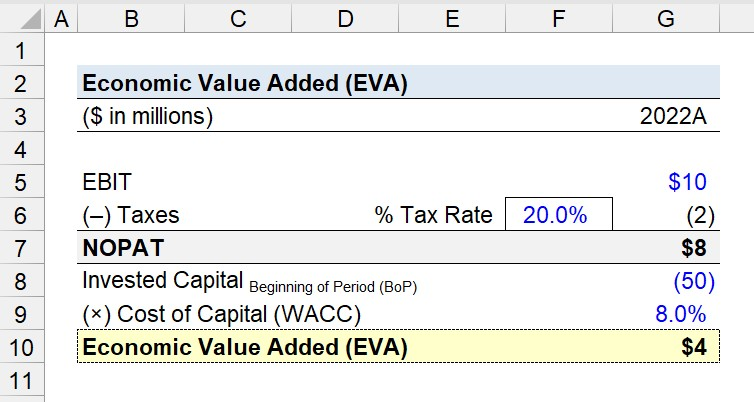Welcome to Wall Street Prep! Use code at checkout for 15% off.Guide to Understanding Economic Value Added (EVA)## How to Calculate Economic Value Added (EVA)?

The economic value added (EVA), or “economic profit,” measures the surplus in profitability generated by a project in excess of the minimum spending required to fund the cost of the project.

By undertaking a project, companies expect the income produced to not only the capital cost (i.e. break-even), but also to have a surplus in profit, which reflects the concept of economic value add (EVA).

The economic value added (EVA) is the excess profit generated beyond the capital cost of a project.

Conceptually, the economic value added (EVA) is the “spread” between the return on invested capital (ROIC) of a firm and its cost of capital (WACC), multiplied by its total invested capital.

The more residual income created by a project, the more economic value generated by the project that is above its cost of capital (WACC).

If the projects undertaken by a company consistently produce profits in excess of the capital cost, shareholders will perceive the decision-making and capital allocation strategy of the management team more positively.

The economic value added (EVA) is also a method to analyze the profitability of corporations.

Instead of subtracting the capital cost from the profit earned by a project, the company’s net operating profit after taxes (NOPAT) is adjusted for the cost of all capital employed to generate those profits.

The cost of capital (WACC) is inclusive of the cost of debt (kd) and the cost of equity (ke), so all stakeholders in the capital structure are represented in the metric.

While the return on investment (ROI) is the percent return earned on the original investment, the economic value added (EVA) is the surplus income a project or company generates in excess of its capital costs.

## What is a Good Economic Value Added?

On behalf of shareholders, excess profits retrieved on a project represent the creation of shareholder wealth.

• Positive Economic Value Added (EVA) → Value-Creation
• Negative Economic Value Added (EVA) → Value-Destruction

Since economic value added (EVA) measures the value creation of a corporation’s operations, the metric is a tool to measure operating performance and profitability from its core business activities.

The more excess economic value added (EVA) produced by a specific project or company, the more economic profits reaped and the higher the return on investment (ROI).

Thus, the EVA quantifies the economic profit generated by a company relative to its capital investment.

Often, the EVA is determined to serve as a standardized benchmark for comparability, where the performance of industry peers (or those operating in adjacent industries) is compared.

## Economic Value Added Formula (EVA)

The formula to calculate the economic value added (EVA) is the after-tax operating profit minus the total capital invested.

Economic Value Added (EVA) = NOPAT  (Cost of Capital × Invested Capital)

Where:

NOPAT = EBIT × (1  Tax Rate)
Cost of Capital (WACC) = Cost of Equity (Equity Weight) + After-Tax Cost of Debt (Debt Weight)
Invested Capital = Shareholders’ Equity + Beginning Long-Term Debt

In contrast, the economic value added (EVA) can be analyzed using the return on invested capital (ROIC) metric.

Economic Value Added (EVA) = (Return on Capital  Cost of Capital) × Invested Capital

## Economic Value Added Calculator (EVA)

We’ll now move to a modeling exercise, which you can access by filling out the form below.#### Excel TemplateSubmitting...

## Economic Value Added Calculation Example (EVA)

Suppose the FP&A team at a publicly traded corporation is tasked with calculating the economic value added (EVA) of a project in 2022.

By performing the analysis, the FP&A team is trying to confirm the project was indeed profitable and created positive economic value for their shareholders.

In 2022, the first year in which the project was fully operational, \$10 million in EBIT was generated.

• EBIT = \$10 million

If we assume the applicable tax rate was 20.0%, the net operating profit after tax (NOPAT) is \$8 million.

• NOPAT = \$10 million × (1 – 20.0%) = \$8 million

The total amount of capital invested at the beginning of the period was \$50 million, while the cost of capital (WACC) – the weight-adjusted expected rate of return – is 8.0%.

• Invested Capital, Beginning of Period (BoP) = (\$50 million)
• Cost of Capital (WACC) = 8.0%

The economic value added (EVA) can be calculated by determining the product of invested capital and the cost of capital (WACC), and then subtracting the amount from NOPAT to arrive at \$4 million.

In conclusion, the \$4 million in economic value added (EVA) represents the excess profit remaining from the project, implying the project created positive value.Step-by-Step Online Course

### Everything You Need To Master Financial Modeling

Enroll in The Premium Package: Learn Financial Statement Modeling, DCF, M&A, LBO and Comps. The same training program used at top investment banks.